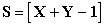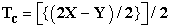﻿ Rapid Calculation of the Number of π-bonds, σ-bonds, Single and Triple Bonds in Aliphatic Unsaturated Open Chain and Cycloalkynes

### Rapid Calculation of the Number of π-bonds, σ-bonds, Single and Triple Bonds in Aliphatic Unsaturate...

Arijit Das, Suman Adhikari, Debapriya Pal, Bijaya Paul, R. Sanjeev, V. JagannadhamOPEN ACCESSPEER-REVIEWED

## Rapid Calculation of the Number of π-bonds, σ-bonds, Single and Triple Bonds in Aliphatic Unsaturated Open Chain and Cycloalkynes

Arijit Das1,, Suman Adhikari1, Debapriya Pal2, Bijaya Paul3, R. Sanjeev4, V. Jagannadham5

1Department of Chemistry, Govt. Degree College, Dharmanagar, Tripura(N), India

2Department of Science, Netaji Vidyapith Institute, Unakoti, Tripura, India

3Department of Chemistry, Tripura University, Suryamaninagar, Tripura(W), India

4Department of Chemistry, Avanthi Degree and PG College, Hyderabad, India

5Department of Chemistry, Osmania University, Hyderabad, India

### Abstract

Prediction of number of π-bonds, σ-bonds, single and triple bonds of aliphatic unsaturated open chain and cycloalkynes is a vitally important tool for students of chemistry at undergraduate and graduate level for solving different kinds of problems regarding different chemical reactions. In this manuscript, we try to present a simple and innovative method for easy calculation of number of π-bonds, σ-bonds, single and triple bonds with the help of completely 8 (eight) new formulae.

• Das, Arijit, et al. "Rapid Calculation of the Number of π-bonds, σ-bonds, Single and Triple Bonds in Aliphatic Unsaturated Open Chain and Cycloalkynes." World Journal of Chemical Education 2.1 (2014): 1-3.
• Das, A. , Adhikari, S. , Pal, D. , Paul, B. , Sanjeev, R. , & Jagannadham, V. (2014). Rapid Calculation of the Number of π-bonds, σ-bonds, Single and Triple Bonds in Aliphatic Unsaturated Open Chain and Cycloalkynes. World Journal of Chemical Education, 2(1), 1-3.
• Das, Arijit, Suman Adhikari, Debapriya Pal, Bijaya Paul, R. Sanjeev, and V. Jagannadham. "Rapid Calculation of the Number of π-bonds, σ-bonds, Single and Triple Bonds in Aliphatic Unsaturated Open Chain and Cycloalkynes." World Journal of Chemical Education 2, no. 1 (2014): 1-3.

 Import into BibTeX Import into EndNote Import into RefMan Import into RefWorks

### 1. Introduction

The number and types of bonds in open chain and cycloalkynes having complex molecular formula is a Herculean task. Keeping this in view, a rapid method has been proposed for the calculation of number of π-bonds, σ-bonds, single and triple bonds with the help of following 08 (eight) completely new formulae for certain aliphatic unsaturated open chain and cycloalkynes.

Earlier eight new innovative methods including sixteen new formulae have been introduced on the easy prediction of ‘Bond-Order of mono and diatomic homo and heteronuclear molecules or ions’, ‘Bond-order of oxide based acid radicals’, ‘Hybridization’, ‘IUPAC nomenclature of spiro and bicyclo compounds, ‘spin multiplicity value calculation and prediction of magnetic properties of diatomic hetero nuclear molecules and ions, ‘Aromaticity’ and ‘magnetic properties of homo and hetero nuclear diatomic molecules and ions [1-7].

The present study involves eight formulae by just manipulating the number of carbon and hydrogen atoms by using some factors.

We think it would go a long way to help the students of organic chemistry and applied chemistry also who would choose the subject as their carrier. Experiment in vitro on 100 students show that by using these new methods strike rate is 1Q/30secs.

On the basis of this, we can strongly recommend that by using these new formulae the students can calculate number of π-bonds, σ-bonds, single and triple bonds for aliphatic unsaturated open chain and cycloalkynes.

### 2. Result and Discussion

2.1. Open Chain Aliphatic Alkynes
2.1.1. Calculation of π-bonds (P)

In the first case, we have to count the number of carbon atoms (X) and the number of hydrogen atoms (Y) in a given unsaturated hydrocarbon containing triple bonds. The formula to calculate the number of π bonds for an aliphatic open chain alkyne, where there is one or more than one triple bonds isWhere, X = number of carbon atoms; Y = number of hydrogen atoms and P = number of π bonds.

E.g.: In C16H30, X = 16, Y = 30, therefore P = [{(2X-Y)/2} + 1] = [{(2 x 16 – 30)/2} +1] = 1 + 1 = 2 number of π bonds.

2.1.2. Calculation of σ-bonds (S)

In the first case, we have to count the number of carbon atoms (X) and the number of hydrogen atoms (Y) in a given unsaturated hydrocarbon containing triple bonds. The formula to calculate the number of σ bonds for an aliphatic open chain alkyne, where there is one or more than one triple bonds isWhere, X = number of carbon atoms; Y = number of hydrogen atoms and S = number of σ bonds.

E.g.: In C16H30, X = 16, Y = 30, therefore, S= [X+Y-1] = [16+30-1] = 45 numbers of σ bonds.

2.1.3. Calculation of Single bonds (A)

The total number of single bond for an aliphatic open chain alkyne, where there is one or more than one triple bonds is A = [{(2X+5Y)/2} - 3]/2

Where, A = number of single bonds, X = number of carbon atoms and Y = number of hydrogen atoms.

E.g.: In C16H30, X = 16, Y = 30, therefore, A = [{(2X+5Y) / 2} - 3]/2 = [{(2x16+5x30)/2}-3]/2=[91-3]/2 = 44 numbers of single bonds.

2.1.4. Calculation of Triple Bonds (T)

In the first case, we have to count the number of carbon atoms (X) and the number of hydrogen atoms (Y) in a given unsaturated hydrocarbon containing triple bonds. The formula to calculate the number of triple bonds for an aliphatic open chain alkyne, where there is one or more than one triple bonds isWhere, X = number of carbon atoms; Y = number of hydrogen atoms and T = number of triple bonds.

E.g.: In C16H30, X = 16, Y = 30, therefore, T = [{(2X-Y)/2} + 1]/2 = [{(2 x 16 – 30)/2} +1]/2 = 2/2 = 1 triple bond.

#### Table 1.Download asPowerPoint Slide

Veiw figureView current table in a new window

2.2. Cycloalkynes
2.2.1. Calculation of π-bonds (Pc)

In the first case, we have to count the number of carbon atoms (X) and the number of hydrogen atoms (Y) in the given unsaturated cycloalkyne. The formula to calculate the number of π bonds for an aliphatic cycloalkyne isWhere, X = number of carbon atoms; Y = number of hydrogen atoms and Pc = number of π bonds in the cycloalkyne system.

E.g.: In Cycloheptyne (C7H10), X =7, Y = 10, therefore Pc = (2x7-10)/2 = 2 number of π bonds.

2.2.2. Calculation of σ-bonds (Sc)

In the first case, we have to count the number of carbon atoms (X) and the number of hydrogen atoms (Y) in the given unsaturated cycloalkyne. The formula to calculate the number of σ bonds for an aliphatic cycloalkyne isWhere, X = number of carbon atoms; Y = number of hydrogen atoms and Sc = number of sigma bonds (σ-bonds) in cyclo alkyne system.

E.g.: In Cycloheptyne (C7H10), X =7, Y = 10, therefore Sc = (7+10) = 17 number of σ bonds.

2.2.3. Calculation of Single bonds (Ac)

The total number of single bond for an aliphatic cyclo alkyne isWhere, Ac = number of single bonds in cycloalkyne, X = number of carbon atoms and Y = number of hydrogen atoms.

E.g.: In Cycloheptyne (C7H10), X = 7, Y = 10, therefore, Ac = [{(2X+5Y)/2}]/2 = [{(2x7+5x10)/2}]/2=32/2 = 16 numbers of single bonds.

2.2.4. Calculation of Triple bonds (T)

In the first case, we have to count the number of carbon atoms (X) and the number of hydrogen atoms (Y) in a given unsaturated cyclo system containing triple bond. The formula to calculate the number of triple bond isWhere, X = number of carbon atoms; Y = number of hydrogen atoms and Tc = number of triple bond.

E.g.: In Cycloheptyne (C7H10), X =7, Y = 10, therefore, Tc = [{(2X-Y)/2}]/2 = [{(2 x 7 – 10)/2}]/2 = 2/2 = 1 triple bond.

### 3. Conclusions

In conclusion here we approach a new rapid innovative method on easier calculation of number of π-bonds, σ-bonds, single and triple bonds for aliphatic unsaturated open chain and cycloalkynes. This new method is very helpful to undergraduate and graduate level students of chemistry. By using these methods we can easily predict nature of bonds in alkyne system in a very simple and metabolic way.

### Acknowledgement

The corresponding author, Dr. Arijit Das, would be grateful to Dr. S. Rakshit, Principal, Govt. Degree College, Dharmanagar, Tripura (N), Tripura, India, for giving the opportunity to carry out the research work.

### References

  Das Arijit, ‘New Innovative methods for prediction of Bond order of mono and diatomic molecules, ions and also acid radicals in a very short time’, Ind. J. of Applied Research, 03(07), p 114, 2013.In article  Das Arijit, ‘New Innovative Methods for Prediction of Hybridization State in a Very Short Time’, Ind. J. of Applied Research, 03(07), p 594, 2013.In article  Das Arijit, ‘New Innovative Methods for IUPAC Nomenclature of Bicyclo and Spiro Compounds in Organic Chemistry’, Ind. J. of Applied Research, 03 (07), p 596, 2013.In article  Das Arijit, ‘New Innovative Methods for Determination of Spin Multiplicity, Spin State and Magnetic Properties of Diatomic Hetero Nuclear Molecules or Ions in a Very Short Interval of Time’ Ind. J .of Applied Research, 03 (08), p 67, 2013.In article  Das Arijit, ‘New Innovative methods for prediction Bond order of mono and diatomic molecules or ions having total number of (1-20) es in a very short time’, Ind. J. of Applied Research, 03(09), p 63, 2013.In article  Das Arijit, Adhikari Suman, Paul Bijaya, Sanjeev R. and Jagannadham V., ‘A rapid and innovative method for the identification of aromatic and anti-aromatic nature of organic compounds’, World Journal of Chemical Education, 01(01), p 6, Sept-2013.In article  Das Arijit, ‘A rapid and innovative method for the easy prediction of Magnetic behavior of homo and hetero nuclear mono and diatomic molecules or ions without MOT’, Ind. J .of Applied Research, 03(10), 2013.In article